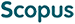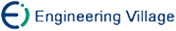周明华, 汪国昭. 基于遗传算法的B样条曲线和Bézier曲线的最小二乘拟合[J]. 计算机研究与发展, 2005, 42(1): 134-143.
 引用本文: 周明华, 汪国昭. 基于遗传算法的B样条曲线和Bézier曲线的最小二乘拟合[J]. 计算机研究与发展, 2005, 42(1): 134-143.Zhou Minghua, Wang Guozhao. Genetic Algorithm-Based Least Square Fitting of B-Spline and Bézier Curves[J]. Journal of Computer Research and Development, 2005, 42(1): 134-143.
 Citation: Zhou Minghua, Wang Guozhao. Genetic Algorithm-Based Least Square Fitting of B-Spline and Bézier Curves[J]. Journal of Computer Research and Development, 2005, 42(1): 134-143.## Genetic Algorithm-Based Least Square Fitting of B-Spline and Bézier Curves

• 摘要: 考虑用B样条曲线拟合平面有序数据使得最小二乘拟合误差最小.一般有两种考虑，一种是保持B样条基函数的节点不变，选择参数使得拟合较优.参数的选择方法包括均匀取值、累加弦长法、centripetal model、Gauss-Newton迭代法等.另一种则是先确定好参数值(一般用累加弦长法)，然后再用某一算法计算出节点，使得拟合较优.同时把两者统一考虑，用遗传算法同时求出参数、节点使得拟合在最小二乘误差意义下最优.与Gauss-Newton迭代法、Piegl算法相比，本方法具有较好的鲁棒性(拟合曲线与初始值无关)、较高的精度及控制顶点少等优点.实验结果说明采用遗传算法得到的曲线逼近效果更好.用遗传算法对Bézier曲线拟合平面有序数据也进行了研究.

Abstract: To obtain a good approximation for data fitting with a spline, parameters and knots have to be treated as variables frequently. There are two kinds of considerations. The first is to choose parameters with which the fitting are better while the knots of the B-spline bases are in a fix. The choices of parameters include uniform parameterization, cumulative chord length parameterization, centripetal model parameterization and Gauss-Newton approach. The other is to determine parameters in advance (generally cumulative chord length parameterization) and then to compute the knots of B-spline bases by some algorithms such that the fitting becomes more precise. In this paper both the parameters and the knots of the B-spline bases are considered simultaneously by using genetic algorithms such that the fitting B-spline curve to data attains its optimum in the total least squares sense. With this, the parameters and the knots can be appropriately determined simultaneously. The method given in this paper have advantages of robustness (the resulting curve is initial-value-free), better precision and fewer vertexes compared with Gauss-Newton approach and Piegl's algorithm. Two examples of data fitting are given to show that the genetic algorithms-based fitting curves are better in approximation. Fitting Bézier curve to a set of data by using genetic algorithms is also studied./下载:  全尺寸图片 幻灯片
• 分享
• 用微信扫码二维码

分享至好友和朋友圈